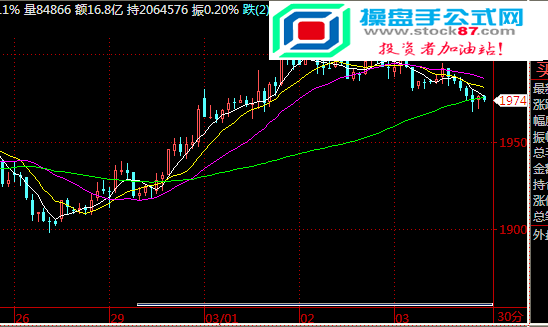﻿ 闪灵交易者策略金字塔软件源码贴图-指标公式网-操盘手公式网当前位置：首页 >> 指标公式 >>  指标公式网

• 相关简介： 今日股市
• 内容标签： 金字塔,软件
• 浏览次数：
• 运行环境： Win10,Win8,Win7,WinXP
• 文章作者： 操盘手公式网
• 文章来源： www.stock87.com
• 加入时间： 2018-09-14 22:41:00
• 解压密码： www.stock87.com（请仔细输入！复制无效！）
•//策略:闪灵交易者系统
//类型:
//版本:1.0
//修订时间:2012.11.24
//DESIGNED BY ROGARZ

//中间变量
INPUT:N1(9,1,100,1),SS(1,1,100);
VARIABLE:该笔盈亏:=0;模拟持仓:=0,模拟开仓价:=0,模拟平仓价:=0,真实系统下单开关:=0;
LC := REF(CLOSE,1);
RSI:=SMA(MAX(CLOSE-LC,0),N1,1)/SMA(ABS(CLOSE-LC),N1,1)*100;//RSI指标默认N1为9
9日收盘价指数平均:REF(EMA(CLOSE,9),1);
19日最高价收盘价平均:REF(EMA(HIGH,19),1);
20日高点:=REF(HHV(H,20),1);
20日低点:=REF(LLV(L,20),1);

//交易条件

//交易系统
//模拟交易模块
IF 开多条件 AND 模拟持仓=0 THEN BEGIN
模拟开仓价:=CLOSE;//记录开仓价
模拟持仓:=1;//模拟持仓为1
END

IF 平多条件 AND 模拟持仓=1 THEN BEGIN
模拟平仓价:=CLOSE;//记录平仓价
该笔盈亏:=模拟平仓价-模拟开仓价;//在模拟交易模块中我们只需计算上一笔交易是赚还是亏，在这里我只计算盈亏最后的点数
模拟持仓:=0;//将全局变量*模拟持仓*初始化为0
IF 该笔盈亏>0 THEN BEGIN
真实系统下单开关:=0;//0代表模拟交易上一笔是赚钱的。
模拟开仓价:=0;//将全局变量*模拟开仓价*初始化为0
模拟平仓价:=0;//将全局变量*模拟开仓价*初始化为0
END
IF 该笔盈亏<=0 THEN BEGIN
真实系统下单开关:=1;//1代表模拟交易上一笔是亏钱的。
模拟开仓价:=0;//将全局变量*模拟开仓价*初始化为0
模拟平仓价:=0;//将全局变量*模拟平仓价*初始化为0
END
END

IF 开空条件 AND 模拟持仓=0 THEN BEGIN
模拟开仓价:=CLOSE;//记录开仓价
模拟持仓:=-1;//模拟持仓为-1
END

IF 平空条件 AND 模拟持仓=-1 THEN BEGIN
模拟平仓价:=CLOSE;//记录平仓价
该笔盈亏:=模拟开仓价-模拟平仓价;//在模拟交易模块中我们只需计算上一笔交易是赚还是亏，在这里我只计算盈亏最后的点数
模拟持仓:=0;//将全局变量*模拟持仓*初始化为0
IF 该笔盈亏>0 THEN BEGIN
真实系统下单开关:=0;//0代表模拟交易上一笔是赚钱的。
模拟开仓价:=0;//将全局变量*模拟开仓价*初始化为0
模拟平仓价:=0;//将全局变量*模拟开仓价*初始化为0
END
IF 该笔盈亏<=0 THEN BEGIN
真实系统下单开关:=1;//1代表模拟交易上一笔是亏钱的。
模拟开仓价:=0;//将全局变量*模拟开仓价*初始化为0
模拟平仓价:=0;//将全局变量*模拟开仓价*初始化为0
END
END

//真实下单模块### 热搜标签浙ICP备17017803号-1